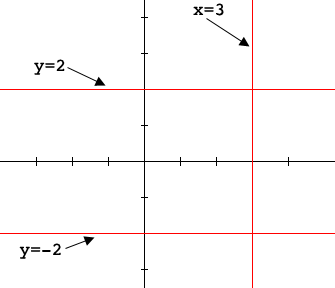SEARCH HOMEMath Central Quandaries & QueriesQuestion from Don: Can one equation with two variables be solved algebraically?Hi Don,

How about $x^2 + y^2 = 0?$ $x = 0, y = 0$ is a solution, but is there another?

Rewrite the equation as $y^2 = -x^{2}. If$x \neq 0$then$x^2$is positive and hence$-x^2$is negative but$y^2$can't be negative. Hence there is no solution with$x \neq 0.$Similarly there is no solution with$y \neq 0.$Hence$x = 0, y = 0$is the only solution of$x^2 + y^2 = 0.$Now look at$xy^2 - 3y^2 - 4x + 12 = 0.$This can be rewritten as$y^2 (x - 3) -4( x - 3) = 0$or$(x-3)(y^2 - 4) = 0.$Thus the product of the two numbers$x - 3$and$y^2 - 4$is zero and hence at least one of them must be zero. Hence the solution of$y^2 (x - 3) -4( x - 3) = 0$is$\left\{ (x,y): x=3, y=2 \mbox{ or } y = -2 \right\}. $Geometrically that's all the points on the lines$x = 3, y = -2$and$y = -2.\$PennyMath Central is supported by the University of Regina and The Pacific Institute for the Mathematical Sciences.